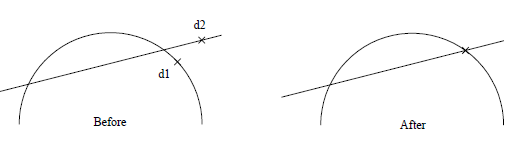## Line Trim

Allows the trimming of the length of any number of lines to their points of intersection with a reference line. The reference line is selected, followed by the lines to be trimmed. When all of the lines to be trimmed are selected, Abort (right mouse button) and confirm. Finally, a location is selected near the end of the lines to be trimmed.

Each selected line has its length altered by its selected endpoint being moved to the point of intersection with the reference line.

1. Lines are regarded as having infinite endpoint extensions to determine the point of intersection.
2. Lines are 3D model entities. Although they may visually appear to intersect in the current view, they may not actually intersect in 3D space.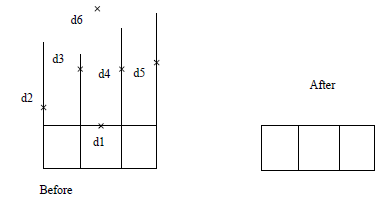Circle   Allows the trimming of the length of any number of lines to their points of intersection with a reference circle. The reference circle is selected, followed by the lines to be trimmed. When all of the trim lines are selected, Abort and confirm. Select a trim location near the end of the lines to trim.

Each selected line has its length altered by its endpoint being moved to the point of intersection with the reference circle.

1. Lines are regarded as having infinite endpoint extensions to determine the point of intersection.
2. Lines and circles are 3D model entities. Although they may visually appear to intersect in the current view, they may not actually intersect in 3D space.
3. An arc may be selected as a reference for trimming a line with this command, but it will be regarded as a circle. Therefore, the line will be trimmed to wherever the imaginary circle intersects.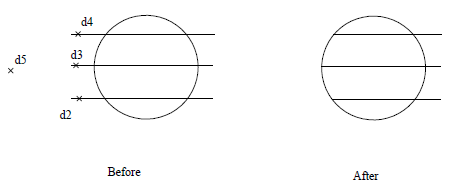Curve  Allows the trimming of the length of any number of lines to their points of intersection with a reference curve.  The reference curve is selected, followed by the lines to be trimmed. When all of the lines to be trimmed are selected, Abort (right mouse button) and confirm. Finally, a location is selected near the end of the lines to be trimmed.

Each selected line has its length altered by its selected endpoints being moved to the point of intersection with the reference curve.

1. Lines are regarded as having infinite endpoint extensions to determine the point of intersection.
2. Lines and circles are 3D model entities. Although they may visually appear to intersect in the current view, they may not actually intersect in 3D space.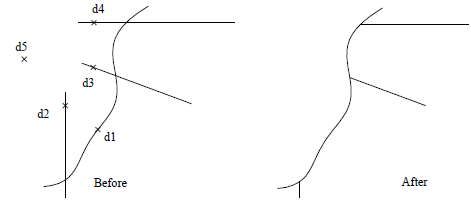Length   Allows the trimming of the length of any number of lines to a specified length. The length is specified, followed by the selection of the lines to be trimmed. When all of the lines to be trimmed are selected,

Abort (right mouse button) and confirm. Finally, a location is selected near the end of the lines to be trimmed.   Each selected line has its length altered to the length specified by moving its selected endpoint.

Lines are regarded as having infinite endpoint extensions, therefore the length of a line may be increased or decreased.

Incr-Len   Allows the trimming of the length of any number of lines by a specified incremental length.

The incremental length is specified, followed by the selection of the lines to be trimmed. When all of the lines to be trimmed are selected, Abort (right mouse button) and confirm. Finally, a location is selected near the end of the lines to be trimmed.

Each selected line has its length altered by its selected endpoint being moved the specified incremental length. A positive incremental length increases the length and a negative incremental length decreases the length.

## Line Divide

The Line Divide command enables the Line Divide sub-menu which allows the division of a line into several shorter lines by specifying:

• a number
• relative to a point location
• relative to its point of intersection with a line, circle or curve
• an incremental length.

Number Allows the division of a line into a specified number of equal length lines. The number of segments is specified followed by the selection of the line to be divided. The selected line is then equally divided into the specified number of lines.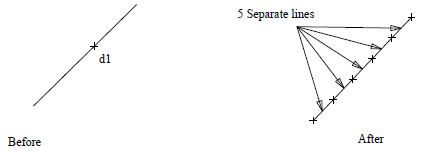Point-Int Allows the division of a line relative to any number of reference point locations.

The line to be divided is selected followed by the reference point location. The reference point location projects a division point through 3D space onto the selected line. The division point of a selected line is the closest point that can be projected onto that line, through 3D space, from the reference point location. (It can be represented as the intersection by the perpendicular projected to that line through 3D space from the reference point location.)

The selected line is then divided into two lines at the division point.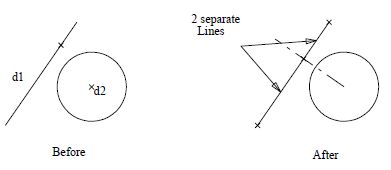Line-Int  Allows the division of a line at its point of intersection with a reference line. The line to be divided is selected followed by the reference line. This results in the selected line being divided at its point of intersection with the reference line into two lines.

This command regards the reference line as having infinite endpoint extensions when determining the division point of the line being divided. Lines are 3D model entities. Although they may visually appear to intersect in the current view, they may not actually intersect in 3D space.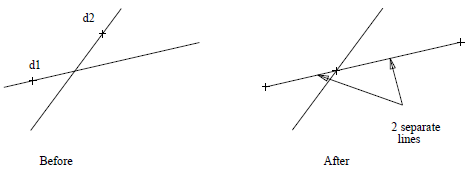Cir-Int  Allows the division of a line at its point of intersection with a reference circle. The line to be divided is selected first, followed by the reference circle near the intersection point. This results in the line being divided at its point of intersection with the reference circle into two lines.

Lines and circles are 3D model entities. Although they may appear to intersect in the current view, they may not actually intersect in 3D space. An arc may be selected as a reference for dividing a line with this command, but it will be regarded as a circle. Therefore, the line will be divided wherever the imaginary reference circle intersects it.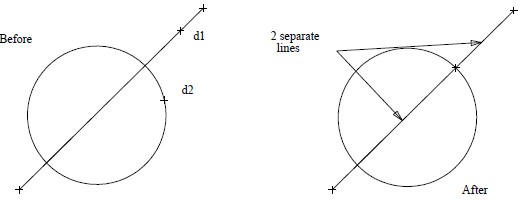Length Allows the division of a line at a specified length from one of its endpoints. The length is specified followed by the selection of the line to be divided near one of its endpoints. This results in the selected line being divided into two lines at the specified length measured from the selected endpoint.

## Circle Divide

The Circle Divide command enables the Circle Divide sub-menu which allows a circle to be divided into several arcs by specifying:

• a number • relative to a point location
• relative to its point of intersection with a line, circle or curve
• or an incremental angle.

Number   Allows the division of a circle into a specified number of equal angled arcs. The number is specified followed by the selection of the circle to be divided. The selected circle is then equally divided into the specified number of arcs beginning at the start point of the circle.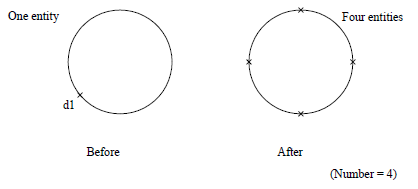Point-Int  Allows the division of a circle into two arcs by specifying relative to a reference point location. The circle to be divided is selected followed by the selection of the reference point location. This reference point location projects a division point through 3D space onto the selected circle. This division point is the

closest point that can be projected onto that circle through 3D space from the reference point location. (It can be represented as an intersection by the shortest perpendicular projected to that circle through 3D space from the reference point location.)

The two resultant arcs of the divided circle have angles subtending from the start/endpoint of the original circle to the division point.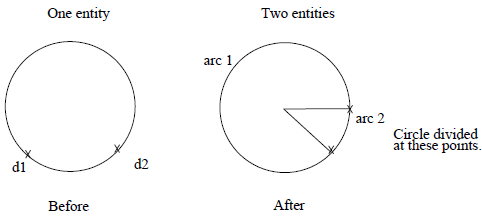Line-Int   Allows the division of a circle into two arcs by specifying relative to its point of intersection with a reference line. The circle to be divided is selected followed by the selection of the reference line near the required point of intersection. This results in the circle selected for division having a division point at its point of intersection with the reference line.

The two resultant arcs of the divided circle have angles subtending from the start/endpoint of the original circle to the division point.

1. This command regards the reference line as having infinite endpoint extensions when determining the division point of the circle.
2. Lines and circles are 3D model entities. Although they may appear to intersect in the current view they may not actually intersect in 3D space.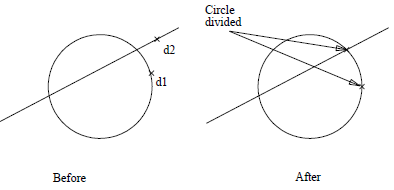Cir-Int   Allows the division of a circle into two arcs by specifying relative to its point of intersection with a reference circle. The circle to be divided is selected near the required point of intersection followed by the selection of the reference circle. This results in the circle selected for division having a division point at its point of intersection with the reference circle. The two resultant arcs of the divided circle have angles subtending from the start/endpoint of the original circle to the division point.

1. Circles are 3D model entities. Although they may appear to intersect in the current view, they may not actually intersect in 3D space.
2.An arc may be selected as a reference for dividing circles with this command but it will be regarded as a circle. Therefore, the circles will be divided wherever the imaginary reference circle intersects them.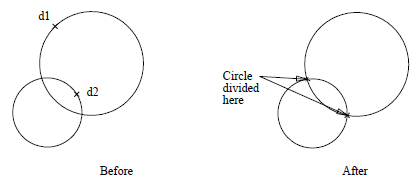Incr-Angle   Allows the division of any number of circles by specifying an incremental angle. The incremental angle and the number of divisions are specified, followed by the selection of the circles to be divided. The start/endpoints of these circles from which the incremental angles are measured are then specified by selecting a point location.

This results in each selected circle having division points defined by the specified number of incremental angles calculated from its start/endpoint in a direction around the circumference dependent upon the direction of that circle. The resultant arcs of each divided circle have endpoints corresponding to the division points of that circle.

The direction of a circle is dependent upon how it was originally inserted. This can be changed using the

Group Change-Dir command.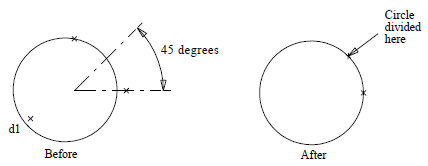## Arc Divide

The Arc Divide command enables the Arc Div sub-menu which allows an arc to be divided into several arcs by specifying:

• a number
• relative to a point location
• relative to its point of intersection with a line, circle or curve
• an incremental angle.

Number  Allows the division of an arc into a specified number of equal angled arcs. The number is specified followed by the selection of the arc to be divided. The selected arc is divided into the specified number of arcs.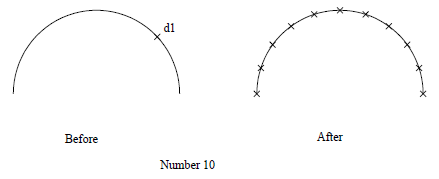Point-Int  Allows the division of an arc, into two arcs, by specifying relative to a reference point location. The arc to be divided is selected, then the reference point location. This reference point location projects a division point, through 3D space, onto the selected arc. This division point is the closest point that can be projected onto that arc, through 3D space, from the reference point location. (It can be represented as an intersection by the shortest perpendicular projected to that arc, through 3D space, from the reference point location.)Line-Int  Allows the division of an arc, into two arcs by specifying relative to its point of intersection with an existing line. The arc to be divided is selected near the required point of intersection followed by the selection of the reference line. This point of intersection is the division point of the arc resulting in the arc being divided into two arcs at its division point.

1. This command regards the reference line as having infinite endpoint extensions when determining the division point of the arc.
2. Lines and arcs are 3D model entities. Although they may appear to intersect in the current view, they may not actually intersect in 3D space.